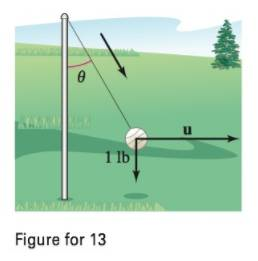Chapter 11, Problem 13PS

Chapter
Section
Textbook Problem

# Tetherball A tetherball weighing 1 pound is pulled outward from the pole by a horizontal force u until the rope makes an angle of θ degrees with the pole (see figure).(a) Determine the resulting tension in the rope and the magnitude of u when θ = 30 ∘ .(b) Write the tension T in the rope and the magnitude of u as functions of θ . Determine the domains of the functions.(c) Use a graphing utility to complete the table. θ 0° 10° 20° 30° 40° 50° 60° T ‖ u ‖ (d) Use a graphing utility to graph the two functions for 0 ∘ ≤ θ ≤ 60 ∘ .(e) Compare T and ‖ u ‖ as θ increases.(f) Find (if possible) lim θ → π / 2 − T   and   lim θ → π / 2 + ‖ u ‖ Are the results what you expected? Explain.(a)

To determine

To calculate: The rope’s tension and magnitude of the vector u when θ=30.

Explanation

Given:

A tetherball that weights 1 pound is pulled outward from the pole by a horizontal force u until the rope makes an angle of θ degrees with the pole.

Formula used:

The horizontal force is found by,

u=(sinθ)T

Tension is found by,

weight=Tcosθ

Calculation:

At, θ=30,

wTcos(30)=01=Tcos(30)

(b)

To determine

To calculate: The domain of the function as function of θ.

(c)

To determine

To fill: The following table using a graphing calculator.

θ0°10°20°30°40°50°60°Tu

(d)

To determine

To graph: The two functions T=secθ and u=tanθ for 0θ60 using TI-83.

(e)

To determine
The comparison between T and u as θ increases.

(f)

To determine

To calculate: The limθπ2T and limθπ2u if possible then explain it.

### Still sussing out bartleby?

Check out a sample textbook solution.

See a sample solution

#### The Solution to Your Study Problems

Bartleby provides explanations to thousands of textbook problems written by our experts, many with advanced degrees!

Get Started